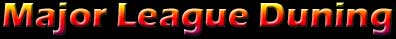A Collection of Helpful or Amusing Javascripts

 Automotive Spring Rate Calculator Calculates Primary Spring Rate when using dual-rate springs Airflow needed Estimate airflow needed for horsepower Horsepower Estimate Horsepower from intake airflow Carburetor Size Calculates required carburetor size Fuel injector flow vs. pressure Calculates fuel injector flow when changing fuel pressure Compression Ratio Calculates Compression Ratio HP gain from boost Estimate HP gain from boost pressure 1/4 Mile MPH Calculate 1/4 mile MPH from vehicle horsepower and weight 1/4 Mile ET Calculate 1/4 Mile ET from horsepower 1/4 Mile Horsepower Calculate Vehicle Horsepower from 1/4 mile MPH 1/4 Mile Horsepower Calculate Vehicle Horsepower from 1/4 mile ET MPH from gear ratios Estimate MPH based on gear ratios and tire size Engine Size Calculates engine size/bore/stroke/rod ratio
 Scientific, Mathematic and Misc. Scripts General Conversions lb to Kg, ft/lb to NM, HP to KW, MPH to KPH... Periodic Table A periodic table of the elements Temperature Converter Converts between Celsius and Fahrenheit Coin Flipper Randomly flips a coin Text Encrypter Encrypts and decrypts text into binary form Quadratic  solver Solves that pesky quadratic formula Roman Numeral Converter Converts regular numbers into Roman Numerals 2nd order Polynomial Calculates the real or complex roots of a 2nd order polynomial Acceleration Equivalents Converts acceleration units Area Equivalents Converts units of area Cylinder Volume Calculates the volume of a cylinder Base Converter Converts input between binary, ternary, quintal, octal, decimal, and hexadecimal bases Degrees to Radians Converts degrees to radians Energy Equivalents Converts Energy equivalents Your age in dog years Calculates your age in dog years Wavelength Calculates wavelength of a frequency WindChill Calculates wind chill temperature Your Japanese Name Tells you how to pronounce your name in Japanese Polygon Figures characteristics of polygons Prime Number Determines if input is prime Pythagorean Theorem Enter a and b and the script solves for c Length Equivalents Converts units of length Mass Equivalents Converts units of mass Atmospheric Calculations Provides atmospheric calculations based on altitude and speed LCM and GCD Calculate the Least Common Multiple and Greatest Common Divisor of two numbers Factors Determines factors of a number Kinetic Energy Calculates Kinetic energy Driving Distance Estimates Driving Distance between cities Airport Distance Distances between major airports Morse Code Converts typed words into Morse Code Power Equivalents Converts Power units Pressure Equivalents Convert Pressure units